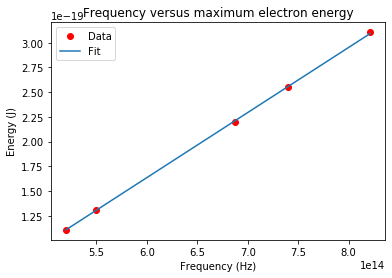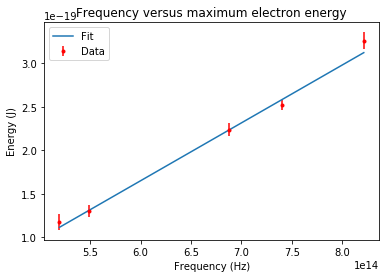# Data Fitting¶

One of the most common data analysis problems and, one you will frequently encounter in a physics laboratory is fitting a curve to a set of data points. Suppose you have a set of $N$ data points $\{x_i, y_i\}$ and that you wish to fit the data to the function $$y(x) = a x + b,$$ where the $a$ and $b$ are adjustable parameters that give the "best fit." This page just describes the programming that needs to be done to find the best fit parameters $a$ and $b$ and their associated uncertainties. We will use data from an experiment to measure Planck's constant to illustrate the process. You can read more about what constitues the "best fit" on the Scientific Computing Toolbox website or refer to one of the books referenced below.

## Finding Planck's Constant¶

In 1921, Einstein was give the Nobel Prize "for his services to Theoretical Physics, and especially for his discovery of the law of the photoelectric effect." His law predicts that when light of frequency $f$ shines on a metal electrons will be ejected from the metal with a maximum kinetic energy $$K_{max} = h f - \phi,$$ where $h$ is Planck's constant and $\phi$ is the work function of the metal. If we can measure the maximum kinetic energy of the electrons for light of several frequencies we should be able to determine Planck's constant and the work function by doing a linear fit. The slope of the line will give Planck's constant and the intercept will give the work function.

### Fitting without uncertainties¶

The table below shows some data for a photoelectric effect experiment. There are no uncertanty estimates for the data. There is always some uncertainty in any measurement, but sometimes it's difficult to estimate. That's the case with these data.

$f$ (Hz) $K_{max}$ (J)
8.213×1014 3.107×10-19
7.402×1014 2.551×10-19
6.876×1014 2.200×10-19
5.491×1014 1.306×10-19
5.196×1014 1.110×10-19

Before we can fit the data, we must put it in a computer readable text file. You can use Atom or some other text editor to create the file. The file must consist of two columns separated by spaces as shown below.

8.213e+14   3.107e-19
7.402e+14   2.551e-19
6.876e+14   2.200e-19
5.491e+14   1.306e-19
5.196e+14   1.110e-19

You can just copy and paste the above text into a text file and save the file with the name data_noerror.txt. Once you have this file you will be ready to go through the steps needed to do a linear fit. Start by begining a Spyder or IPython session so you can follow along. Don't forget to save your program in the same folder as the data_noerror.txt file. (If you like you can download the fitting.zip file. It contains the data files, the fitting programs, and this page's Jupyter Notebook.)

The first step is to import the needed Python modules.

In :
from pylab import *
from scipy.optimize import curve_fit


The first line above is the familar command for importing the entire pylab module. The second line imports a single function curve_fit() from the scipy.optimize module.

Next we have our program read the data from the data_noerror.txt file.

In :
freq,Kmax=loadtxt('data_noerror.txt',unpack=True)


This command reads the data from the file data_noerror.txt and loads the first column in the file into the freq array and the second column into Kmax. The unpack=True argument is needed to load the columns properly. (See the SciPy documentation if you want to understand exactly what unpack=True argument does.)

Now we need to define the function we wish to fit. The curvefit() function will actually fit any arbitrary function. When we run curve_fit() we must pass it the name of a fitting function that we must write. In this case we want to fit our data to a straight line. In Python we define functions with the def command followed by the name of the function and then the function's arguments. All indented lines after the definition line are included in the function. Below is the definition of our linear function.

In :
def linearFunc(x,intercept,slope):
y = intercept + slope * x
return y


The return y statement tells Python to return the value of y whenever the function is called. For example:

In :
linearFunc(1,2,3)

Out:
5

You can give your function any name you like. I called it linearFunc.

The next step is to actually do the fit using curvefit(). We must pass curvefit() the name of the function to fit, the horizontal axis data, and the vertical axis data. The program returns some arrays containing the best fit parameters and the covariance matrix. We will use the covariance matrix to determine the uncertainites in the slope and intercept of the best fit line.

In :
a_fit,cov=curve_fit(linearFunc,freq,Kmax)


The next two lines assign the best-fit parameters returned by the curve_fit() to the variable inter and slope.

In :
inter = a_fit
slope = a_fit


Next, the uncertainties in the intercept and slope are computed from the covarience matrix and assigned to the variables d_inter and d_slope.

In :
d_inter = sqrt(cov)
d_slope = sqrt(cov)


The following code is used to plot the data and the fit.

In :
# Create a graph showing the data.
plot(freq,Kmax,'ro',label='Data')

# Compute a best fit from the fit intercept and slope.
Kmax_fit = inter + slope*freq

# Create a graph of the fit to the data.
plot(freq,Kmax_fit,label='Fit')

# Display a legend, label the x and y axes and title the graph.
legend()
xlabel('Frequency (Hz)')
ylabel('Energy (J)')
title('Frequency versus maximum electron energy')

# Save the figure to a file
savefig('noerror_fit.png')

# Show the graph in a new window on the users screen.
show()We will use a the print() command to print the best fit parameters and uncertainties. Here we use Python f-strings to present the result. F-strings are prefixed with an f and allow us to include variable names enclosed in {}. When the string is printed the values for the variables are substituted into the string. An example makes this clearer.

In :
# Display the best fit values for the slope and intercept. These print
# statments illustrate how to print a mix of strings and variables.
print(f'The slope = {slope}, with uncertainty {d_slope}')
print(f'The intercept = {inter}, with uncertainty {d_inter}')

The slope = 6.58375394121593e-34, with uncertainty 4.838930112425639e-36
The intercept = -2.314103516495066e-19, with uncertainty 3.2581297976065808e-21


By default, f-strings usually present the results with more precision than we need, but it's easy to round the numbers to the desired precision. You can learn more about controling the display precision on the Python Documentation website.

This example gives a good result for Planck's constant. It agrees well with the accpeted value.

### Fitting with uncertainties¶

Suppose we had data with estimated uncertainties in $K_{max}$ and wished to take these uncertainties into account in our fit. A table of the data is shown below. A text file with this data called data_error.txt is included in the fitting.zip file.

$f$ (Hz) $K_{max}$ (J) $\delta_{Kmax}$ (J)
8.213×1014 3.261×10-19 9.71×10-21
7.402×1014 2.52×10-19 5.59×10-21
6.876×1014 2.239×10-19 7.08×10-21
5.491×1014 1.299×10-19 6.83×10-21
5.196×1014 1.175×10-19 8.93×10-21

The code is almost identical, but this time we must read the uncertainties from the file, and pass an array containing these uncertainties to curvefit().

It's simple to read the the uncertainties into an array. We just include a variable called d_Kmax on the line calling the loadtxt() function. After the call, the array called d_Kmax contains the uncertainties.

In :
freq,Kmax,d_Kmax=loadtxt('data_error.txt',unpack=True)


We pass the uncertainties to curve_fit by adding the argument sigma=d_Kmax to the function call.

In :
a_fit,cov=curve_fit(linearFunc,freq,Kmax,sigma=d_Kmax)


We get the best fit slope and the uncertainties from a_fit and cov the same way we did before.

In :
inter = a_fit
slope = a_fit
d_inter = sqrt(cov)
d_slope = sqrt(cov)


We use the function errorbar() to plot the data showing error bars on the data points. It works very much like the plot() command. We just have to specify the array containing the uncertainties with an extra argument yerr=d_Kmax. The errorbar() function also requires that we specify the format to be used to display the data points. In this case the argument fmt='r.' will display the data as red dots.

In :
# Create a graph showing the data.
errorbar(freq,Kmax,yerr=d_Kmax,fmt='r.',label='Data')

# Compute a best fit line from the fit intercept and slope.
Kmax_fit = inter + slope*freq

# Create a graph of the fit to the data. We just use the ordinary plot
# command for this.
plot(freq,Kmax_fit,label='Fit')

# Display a legend, label the x and y axes and title the graph.
legend()
xlabel('Frequency (Hz)')
ylabel('Energy (J)')
title('Frequency versus maximum electron energy')

# Save the figure to a file
savefig('error_fit.png')

# Show the graph in a new window on the users screen.
show()Now we can display the numerical result.

In :
print(f'The slope = {slope}, with uncertainty {d_slope}')
print(f'The intercept = {inter}, with uncertainty {d_inter}')

The slope = 6.6560286973775526e-34, with uncertainty 3.5492135520245525e-35
The intercept = -2.343068168255266e-19, with uncertainty 2.395324805774622e-20


When we have estimated uncertainties in the data, then we can estimate the goodness of fit by computing the reduced chi-squared statistic. For a linear fit to a set of $N$ data points $\{x_i,y_i\}$ that have esimated uncertainties in the $y_i$ values of $\{\sigma_i\}$,
$$\chi_r^2 = \frac{1}{N-2}\sum_{i=1}^N \frac{\left(y_i-y(x_i)\right)^2}{\sigma_i},$$ where for a linear fit $y(x) = a + bx$. For a good fit, $\chi_r^2$ should be approximatly equal to one.

In :
chisqr = sum((Kmax-linearFunc(freq,inter,slope))**2/d_Kmax**2)
dof = len(Kmax) - 2
chisqr_red = chisqr/dof
print(f'Reduced chi^2 = {chisqr_red}')

Reduced chi^2 = 1.2633310164062912


In this case, the computed value of $\chi_r^2$ is close to one indicating a good fit. See the Scientific Computing Toobox: Data Fitting page or the references listed at the end of this page for a more complete discussion of the reduced chi-square statistic.

## Summary¶

The code above is all you should need to do linear fits to data. When you're confronted with a new data analysis problem that requires a fit, it is usually faster to modify existing code than to reinvent it every time. I've included two Python scripts, two data files, and the Jupyter Notebook that generated this page in a ZIP file. (Download the fitting.zip file) Feel free to modify the programs and use them when you need to do a linear fit.

A table with a short description of the Python functions used in this tutorial is posted on the Scientific Computing Toolbox: Command Summary Page.

References

1. Bevington and Robinson, Data Reduction and Error Analysis for the Physical Sciences 3rd Edition (McGraw-Hill Education, 2002)
2. Taylor, An Introduction to Error Analysis: The Study of Uncertainties in Physical Measurements 2nd Edition, (University Science Books, 1996)